• 9863020202
• contact@financeseva.in

# Weighted Average Cost of Capital (WACC)

Weighted Average Cost of Capital (WACC) is the average cost to a company of the funds it has invested in the assets of the company. This is composed of a possible combination of debt, preferred shares, common shares and retained earnings. All components of the cost of capital are determined at the current market rates.

%
%
%

%
%

%
%
%

%
%

%

%

#### What is WACC Weighted Average Cost of Capital?

WACC Weighted Average Cost of Capital Definition: Weighted Average Cost of Capital (WACC) is the average cost to a company of the funds it has invested in the assets of the company. This is composed of a possible combination of debt, preferred shares, common shares and retained earnings. All components of the cost of capital are determined at the current market rates.

WACC Weighted Average Cost of Capital Description: When a firm needs to raise capital to buy assets, the capital can be raised from several sources. Investors will by equity shares in the company, investors will buy the company’s bonds, and the company can keep the profits earned through its operations. Any combination of these sources can be used to pay for capital projects. There is a cost to acquire any of these funds, equity investors expect a return on their invested money, and bond holders expect an interest payment to be paid regularly.

If an individual were to borrow money they would be expected to pay interest on that money. If the individual then invested these funds it makes sense that the investment return should exceed the interest cost of the borrowed funds.

This is the same concept that applies to the WACC Weighted Average Cost of Capital of a firm. A firm is expected to make decisions regarding the use capital to benefit shareholders. If the company cannot make a capital investment that will increase returns for shareholders, then it would be expected that the money would be best delivered directly to the shareholders as a dividend.

WACC Weighted Average Cost of Capital then becomes the hurdle rate for capital projects. A capital project is made when a firm purchases assets, with the intention of generating revenue that will exceed the cost of capital. Cash flow methods will be used to determine whether the project will benefit shareholders. The annual cash flows are estimated, and then the discounted at the WACC Weighted Average Cost of Capital rate. If the net present value is positive, then the project will return enough profit to compensate for the cost of capital, as well as a surplus return.

Since WACC Weighted Average Cost of Capital is the required rate of return used to make important decisions regarding a firm’s investments, it is important to estimate the firms cost of capital accurately. We use the WACC Weighted Average Cost of Capital to approve or reject potential projects. If the cost of capital is estimated wrong, then we may be approve projects that will reduce benefits to shareholders, or reject projects that could benefit shareholders.

The WACC Weighted Average Cost of Capital is dependent on the capital structure of a company. The capital structure refers to the amount of each funding source of a company. A firm with a high level of debt relative to its outstanding equity will have a higher weighting of the cost of debt. In this case, if the cost of debt is lower than the cost of equity, the WACC Weighted Average Cost of Capital will be lower as a result.

This is an important concept to understand. Since the cost of capital of a firm is weighted by the amount of each component, adjustments made to the size of each component can change the WACC Weighted Average Cost of Capital. This in turn can change the way that a firm behaves. If the WACC Weighted Average Cost of Capital is lowered, then projects that were previously rejected may now be approved.

It is important to understand this since each new project that a firm engages in will often be funded from a single source. If a firm has a capital structure of 30% debt, and 70% equity, it is unlikely that new projects will be funded from capital raised in the same proportions. As well, the profit from previous projects that is reinvested in new capital projects by the company can also change to weighing of the capital structure. If a firm continually uses retained earnings to fund new capital projects, then the weighting of the equity will increase as the size of retained earnings grows relative to the size of debt. Since the cost of equity is often greater than the cost of debt, this will tend to increase the total WACC Weighted Average Cost of Capital over time. This could limit the future growth of a company if the firm is unable to source new capital projects that will clear the higher WACC Weighted Average Cost of Capital rate.

How to calculate WACC Weighted Average Cost of Capital?
Now that we understand what WACC Weighted Average Cost of Capital is, we now need to understand how to calculate WACC Weighted Average Cost of Capital. To do this, we calculate the cost of each component of WACC Weighted Average Cost of Capital, and then weight each cost relative to its place in the capital structure.

There are two main steps to be taken in order to calculate WACC Weighted Average Cost of Capital. First, we must calculate the cost of each component. Capital can be acquired from four possible sources. Debt is borrowed from banks, insurance companies, governments and through the issuance of bonds to investors. The cost of debt is determined by the interest rate paid to the supplier of these funds.

Preferred shares are similar to debt in that the owners of the preferred shares are paid a regular fixed payment, like an interest payment. Unlike debt, the preferred shares are never repaid, the shares remain outstanding indefinitely, and the dividend payments are made for as long as the shares remain outstanding.

Common equity is acquired by a firm when they sell shares to the public. The holders of the shares expect to be compensated for the use of their capital, as well as the risk they take by investing directly in the company as an owner.

Retained earnings are acquired by a company from the profits it generates through its operations. The retained earnings are assumed to be the equity of the shareholders, that have been reinvested in the company rather than paid out through dividends.

We need to determine what the cost of each component is, along with the acquisition costs, and adjust these by the tax effects of each funding source. There is an important question to answer before determining what the cost of each is.

Do we use the book cost or the market cost of each component? In order to answer this question we need to understand why we use WACC Weighted Average Cost of Capital. WACC Weighted Average Cost of Capital is used to test potential capital projects, we use the WACC Weighted Average Cost of Capital rate to generate the net present value of a project, and we compare the internal rate of return against the WACC Weighted Average Cost of Capital. These projects are forward looking, and are planned against all possible alternatives. The alternative returns that investors can gain are determined by the market at the time the project is proposed.

Although the funds have been acquired at a cost in the past, the funds have already been invested in capital projects at that time. The current project proposal is intended to provide a further return over and above what the investor could otherwise receive if they had these funds to invest elsewhere. So it makes sense to use the market rates associated today, rather than the book costs that reflect the market rates in the past.

The same thing can be said when determining the capital structure. The second step in calculating WACC Weighted Average Cost of Capital is to weight the cost of each component relative to its weight in the capital structure. When determining the capital structure, it is important to value the weighting at market prices and rates. If the stock of a company has increase since, then its weight in the capital structure would have increased relative to the amount of debt. This means that investors have a higher expectation of a return for their equity investment. This reflects the true cost of this funding source for management. When deciding whether to approve a project, management needs to understand the expectations of their shareholders.

The WACC Weighted Average Cost of Capital calculator above will help you determine the WACC Weighted Average Cost of Capital, by calculating the cost of each component, and then weighing it relative to the market value of the capital structure.

WACC Weighted Average Cost of Capital Formula
The WACC Weighted Average Cost of Capital formula is complex, and can be broken into several components. The individual component costs are provided in the following sections.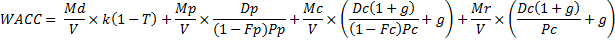WACC Weighted Average Cost of Capital Variables
V=Firm Total Value (Debt + Preferred Shares + Common Equity + Retained Earnings)
Md=Market Value of Debt
Mp=Market Value of Preferred Shares
Mc=Market Value of Common Equity
Mr=Market Value of Retained Earnings
K=Current Market Interest Rate
T=Tax Rate
Dp=Annual Dividends for Preferred Shares
Pp=Market Price of Preferred Shares
Fp=Floatation Costs of Preferred Shares
Dc=Annual Dividends for Common Shares
Pc=Market Price of Common Shares
Fc=Floatation Costs of Common Shares
G=Constant Growth Rate of Common Share Dividends

Cost of Debt Formula
Cost of Debt is the cost to the company for the use of borrowed funds to finance operations.
K=Current Market Interest Rate
T=Tax Rate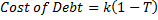Cost of Preferred Shares Formula
Cost of Preferred Shares is the cost to the company for the use of funds generated by selling preferred shares to investors.

D=Annual Dividends
P=Market Price of Shares
F=Floatation Costs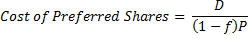Cost of Common Shares Formula
Cost of Common Shares is the cost to the company for the use of funds generated by issuing common shares to investors.

D=Annual Dividends
P=Market Price of Shares
F=Floatation Costs
G=Constant Growth Rate of Dividends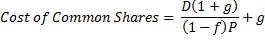Cost of Retained Earnings Formula
Cost of Retained Earnings is the cost to the company for the use of funds retained from previous profits.

D=Annual Dividends
P=Market Price of Common Shares
G=Constant Growth Rate of Dividends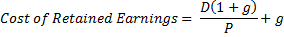How to use the WACC Weighted Average Cost of Capital calculator?
In order to use the WACC Weighted Average Cost of Capital calculator above to calculate the cost of capital, fill in the blank boxes as needed. Each component of WACC Weighted Average Cost of Capital can be completed by inputting the values needed to solve the equation. The cost of each component will then be applied to the capital structure table on the right side of the calculator. Input the market value of each component of the WACC Weighted Average Cost of Capital, and the calculator will provide you with the total WACC Weighted Average Cost of Capital.

Some projects may require new funding, and additional outside funding can change the capital structure, and therefore the WACC Weighted Average Cost of Capital. Input the amount of new funding to be added to the capital structure, as well as the cost (depending on what component is used), and the WACC Weighted Average Cost of Capital will adjust to include the new funding as well.

Enter the cost of capital for each component separately if you do not wish to calculate them using the calculator, as well as the market values for the capital structure, and the manual entry WACC Weighted Average Cost of Capital calculator will provide you with the total WACC Weighted Average Cost of Capital. New funding sources can be included in this section as well, with the total WACC Weighted Average Cost of Capital adjusting to include new funding.

Why is WACC Weighted Average Cost of Capital important?
Investors always have choices about where to invest their capital. Management needs to understand that the shareholders of their company have chosen to invest because they expect a return. Since investors supply funds and companies receive them, the cost of these funds is determined by the expected return of the investors. If management is to engage in activities that will produce this expected return, then any projects that they initiate need to produce at least the expected return. If the management does not have a good understanding of the WACC Weighted Average Cost of Capital of their firm, then they will not be able to make good investment decisions that will benefit the shareholder.

By understanding how the WACC Weighted Average Cost of Capital is calculated, a firms management can choose a capital structure that will provide the highest possible return for their shareholders relative to the perceived risk of the company. It is important to understand that increasing the amount of debt in a company will reduce the WACC Weighted Average Cost of Capital. When this happens, the hurdle rate for capital projects is lowered and projects that were previously rejected may now be approved. But this does not necessarily mean that shareholders will benefit. By increasing leverage, the company has increased the risk to shareholders that returns could be reduced due to the higher amount of interest that need to be paid. It is important to understand the risk expectations of shareholders when making changes to the capital structure.

Why do we use WACC Weighted Average Cost of Capital?
We use WACC Weighted Average Cost of Capital because it reflects the total cost of each funding each project. A firm may decide to fund a potential project entirely with debt. If the cost of debt is lower than the WACC Weighted Average Cost of Capital, it may be approved if the cost of debt is used as the hurdle rate instead of the WACC Weighted Average Cost of Capital rate. It is important for a firm to understand that shareholders do not get to participate in only some projects. The owners of the company will receive the net benefit provided by al projects that are funded by all sources. In order to accurately estimate the benefit to shareholders of pursuing any particular project, management needs to compare it against the total funding cost of all projects. This ensures that when comparing one project against another, that a less profitable project would be approved simply due to a lower cost of capital associated with the project.

What do you use WACC Weighted Average Cost of Capital for?
WACC Weighted Average Cost of Capital is used when performing capital budgeting and cash flow analysis. Any capital project will have an initial investment, should generate additional revenues, and may have additional costs. When analyzing a capital project, annual cash flows are estimated based on the incremental revenues and costs for each year, until the end of the project. These annual amounts are then discounted by the WACC Weighted Average Cost of Capital rate. When we add up the discounted annual cash flow amounts, we have a Net Present Value (NPV). If the net present value is positive, then the project has shown to have provided a return higher than the cost of capital used to fund it. This is a critical test for any capital project. Net present values for different projects will be compared against one another, and many times the highest NPV project will be chosen.

By setting the NPV to zero, and then solving the equation for the interest rate, we develop the Internal Rate of Return (IRR) of a project. This IRR can then be compared against the WACC Weighted Average Cost of Capital rate. If the IRR is higher than the WACC Weighted Average Cost of Capital, the project is said to have satisfied the requirement to provide a return higher than the cost of capital used to fund it.

When comparing the NPV of different projects, the final value can be distorted by the length of the project. A six year project will likely have a higher NPV than a three year project, simply due to the fact that the longer project will produce a higher quantity of benefit. But this does not mean that the longer project is necessarily better. A method for comparing capital projects of different lengths is to use the Equivalent Annual Annuity method. Calculate the payment amount of each NPV as an annuity over the length of time for each project. In this way, the project that produces the highest annual payment can be said to provide the highest return per year.

Discussion of WACC Weighted Average Cost of Capital
There are several issues inherent in calculating the WACC Weighted Average Cost of Capital. The first is that calculating the cost of common equity can be done in several ways. Returns expected by equity investors in a company depend on many things. Investors can always invest in other companies, and so the rate of return that other companies provide to investors becomes important when attracting capital in a firm. If another company can offer investors a greater return on their capital, then it will be difficult for a company to entice investors.

The risk that an investment can reduce in value is an important aspect of making investment decisions that is not explicitly included in WACC Weighted Average Cost of Capital. A high risk company might be able to provide investors a greater return, but the high risk may deter risk averse investors, who will accept a lower rate of return in order to reduce their risk exposure. While the expected returns of a project can be estimated, it is more difficult to estimate risk. If the risk profile for a particular project is higher than the typical risk profile of the company, and WACC Weighted Average Cost of Capital is used to develop NPV and IRR as a test for approval, then the project might be approved even though it increases the risk to the shareholder that their returns can decrease. Since risk is not included in the capital budgeting analysis, it is up to the management of a company to use good judgment and approve projects that fit with the expected risk profile of the company.

There are several ways to calculate the cost of equity of a firm. The most popular are to use the Constant Dividend Growth Rate (Gordon) Model and the Capital Asset Pricing Model (CAM). There are good reasons for both, the Gordon model determines the required rate of return based on the current market price, relative to the dividend payout, and the expected growth rate of those dividends. The CAPM provides us with a required rate of return by comparing the volatility of the company’s stock price to the volatility of the market. This is a good measure as it includes the opportunity cost of the greater market and attempts to factor in the price of risk. The WACC Weighted Average Cost of Capital calculator above uses the Gordon model as it is the most popular method, and avoids comparisons with the market based on volatility, which can be caused by many factors and is not a true determinate of future risk.